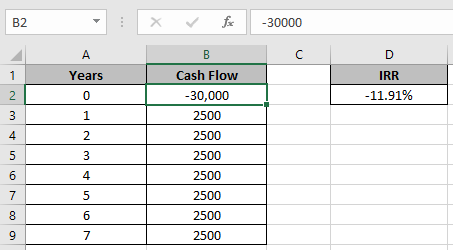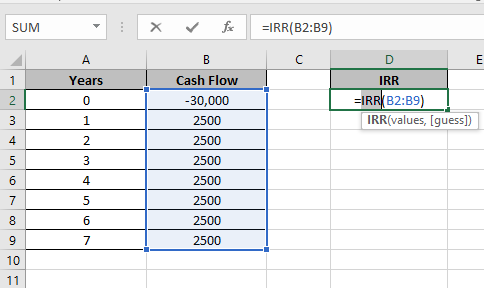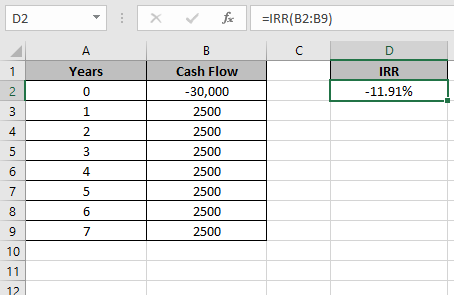# How to use the IRR Function in Excel

In this article, we will learn how to get the Interest rate of return using IRR function in Excel.

IRR function returns the rate of interest of the Cash flow. It takes the argument as array of Cash flow at regular interval unlike XIRR function.
Syntax:

=IRR(Values, [guess])

Values: Array of Cash flow at regular intervals
[guess]: [optional] Expected IRR. Default is .1 (10%)
Let’s learn this through an example shown below.
Here we have Cash flow record paid at the regular interval.Use the formula

=IRR(B2:B9)Press Enter11.91% is the rate of return of the Cash Flow array.
As we took the first amount as negative and others positive so it comes as negative.

Hope you learnt to use IRR function in Excel. Explore more articles here on Accounting. Feel free to state your queries below. We will help you.

Related Articles:

How to Use XIRR function in Excel

How to use the MIRR function in Excel

How to Use RATE Function in Excel

Popular Articles:

50 Excel Shortcuts to Increase Your Productivity

How to use the VLOOKUP Function in Excel

How to use the COUNTIF function in Excel 2016

How to Use SUMIF Function in Excel

Terms and Conditions of use

The applications/code on this site are distributed as is and without warranties or liability. In no event shall the owner of the copyrights, or the authors of the applications/code be liable for any loss of profit, any problems or any damage resulting from the use or evaluation of the applications/code.